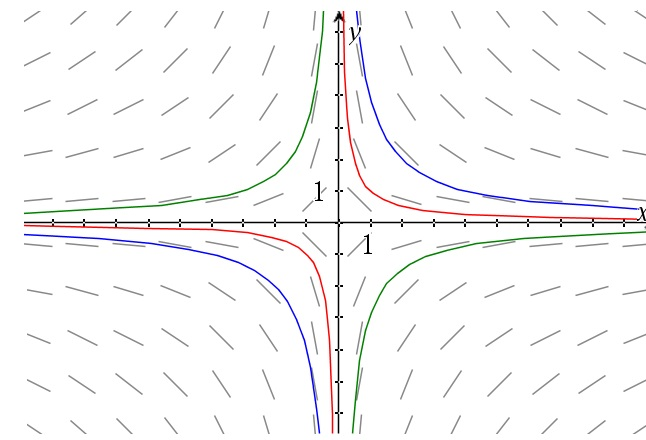# Separable differential equations – A few illustrating examples

Up a level : Differential Equations
Previous page : Separable differential equations
Next page : To find particular solutionsExample 1

Say we have$\frac{{dy}}{{dx}} = \frac{y}{x}$

So the slopes should be equal to the positions? That ought to lines through the origin. Let us see.

We get$\frac{{dy}}{y} = \frac{{dx}}{x}$

Integrating this gives us$\ln |y| = \ln |x| + {C_1}$

or$|y| = {e^{\ln |x| + {C_1}}} = {e^{\ln |x|}}{e^{{C_1}}} = {C_2}|x|$

Yet again, as in the previous page, the signs from the absolute values can be absorbed into the constant to finally give us$y = Cx$

I.e. straight lines through the origin.

Example 2$\frac{{dy}}{{dx}} = - \frac{x}{y}$

Ok, so the slope is now the negative reciprocal of the position. What could that give us? Let us see. We get$ydy = - xdx$

or, after integrating,$\frac{{{y^2}}}{2} = - \frac{{{x^2}}}{2} + {C_1}$

Multiplying through by 2 and rearranging a bit gives us$\frac{{{y^2}}}{2} = - \frac{{{x^2}}}{2} + {C_1}$

In this we should recognise the equation of a circle. Changing the constant to emphasize that we get${x^2} + {y^2} = {r^2}$

Below we can see a graph of the slope field and a few selected solutions.It should hopefully make sense that the derivative is the negative reciprocal of the position for these circles that are concentric around the origin. Thing about the radius, tangent property of circles and that the slopes of perpendicular lines are the negative reciprocals of each other’s.

Example 3$\frac{{dy}}{{dx}} = - \frac{y}{x}$

We get$\frac{{dy}}{y} = - \frac{{dx}}{x}$

or$\int {\frac{{dy}}{y}} = - \int {\frac{{dx}}{x}}$

and thus$\ln |y| = - \ln |x| + {C_1}$

or$\ln |y| = \ln \left| {\frac{1}{x}} \right| + {C_1}$

This gives us$|y| = {e^{\ln \left| {\frac{1}{x}} \right| + {C_1}}} = \left| {\frac{1}{x}} \right|{e^{{C_1}}} = \frac{{{C_2}}}{{\left| x \right|}}$

Yet again we let the constant absorb the signs. This finally gives us$y = \frac{C}{x}$

A slope field with a few particular solutions are shown below.By the way, as you have seen I usually rename the constant using subscripts as it changes throughout the calculations. The final one is usually written without a subscript.

Example 4

If we follow the patterns of the examples we have left to examine$\frac{{dy}}{{dx}} = \frac{x}{y}$

We get$ydy = xdx$

Integration gives us$\frac{{{y^2}}}{2} = \frac{{{x^2}}}{2} + {C_1}$

that gives us${y^2} = {x^2} + C$

A slope field with a few particular solutions are shown below.Yet another example$\frac{{dy}}{{dx}} = \frac{{{x^3}}}{{{y^2}}}$

This gives us${y^2}dy = {x^3}dx$

After integration we get$\frac{{{y^3}}}{3} = \frac{{{x^4}}}{4} + {C_1}$

or${y^3} = \frac{3}{4}{x^4} + C$

A slope field with a few particular solutions are shown below.Up a level : Differential Equations
Previous page : Separable differential equations
Next page : To find particular solutionsLast modified: Apr 1, 2019 @ 16:13